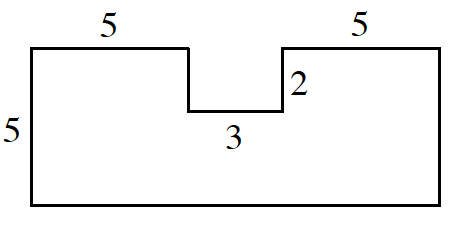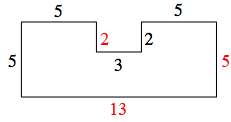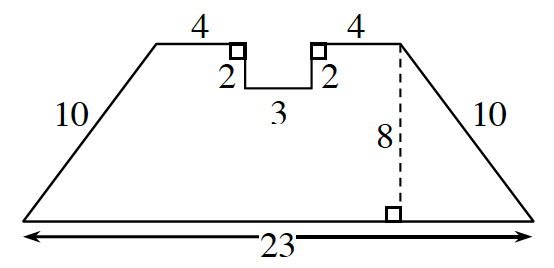### Home > CCAA > Chapter 9 Unit 8 > Lesson CC2: 9.2.1 > Problem9-60

9-60.

Find the area and perimeter of each shape below. Show your steps and work.

1. All angles are right angles.Label the missing sides.One method for calculating the area is to think of the shape as a large rectangle with a small ($2\times3$) rectangle missing.

$\text{Perimeter}=40$ units
$\text{Area}=65-6=59$ units2

1.Area of a trapezoid.

$\text{Area}=\left(\frac{1}{2}\right)(\text{base}_1+\text{base}_2)(\text{height})$